Home » maths » Venn Diagram

Venn Diagram

first of all a swiss mathematician euler gave an idea to represent a set by the points in a closed curve. Later on British mathematician john venn brought this idea to practice. Such the diagrams drawn to represent sets are called venn ealer diagrams or venn diagram.
In venn diagrams the universal set u is represended by points within a rectangle and its sub sets are represented by the point in closed curve (usually circle) with in the rectangle.

SOME TYPES OF REPRESENTATION

U (rectangle) universal Set
A- Set
B- Sub set of A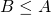are show common element s of set A & set B
intersecting area =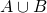suppose u = {1,2,3,4,5,6,7,8,9,10}
Set A = {2,3}
Set B = {6,7,8}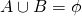OPERATIONS ON SETS

1) Union of sets:
A & B be two sets the union of A & B is the set of all those elements which belong either to A or B or the both A & B.= {x:xA or xB} (A union B)
: xA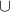B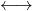xA or xB
And xABxA and xB
As above AB = B (if AB)
AB = A (if BA)

e.g if A = {1,2,3}
B = {1,3,5,7}
Then AB = {1,2,3,5,7}

2) Intersection of sets: A & B be two sets the inter section of A & B is the set of all those elements that belong to both A & b and denoted by AB

Thus AB = {x:xεA and xεB}
Or xABxA and xB
Or AB ≤ A and A∩B ≤ B
e.g if Set A = {1,2,3}
Set B = {1,3,5,7}
Then AB = {1,2,3,5,7}

3) Disjoint set: Two set A & B are said to be disjoint if AB=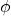If ABthen A and B are said to be intersecting or over capping sets.

4) Difference of sets:

Symmetric difference of two sets:- if A and b be two set s. then symmetric difference of set A and B is A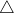b = (A-B)(B-A)
= {x:x AB}
e.g. Set A = {1,2,3,4,6}
Set B = {2,3,4,5,6,7}
Then AB = (A-B)(B-A)
= {1,}(5,7)
AB = {1,5,7}

5) complement of a set– If u is the universal set & A is a set such that A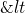U than the complement of set A with respect to set u =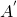oror U-A

Thus == { xu : x A}

e.g- if u = {1,2,3,4,5,6}
A= {3,4}= {1,2,5,6}

Thus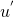=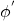= u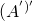= A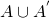= u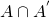=Law of algebra of sets

Managed by MochaHost - Fast & Easy WordPress Hosting